# Text Classification by XGBoost & Others: A Case Study Using BBC News Articles

## Comparative study of different vector space models & text classification techniques like XGBoost and others

In this article, we will discuss different text classification techniques to solve the BBC new article categorization problem. We will also discuss different vector space models to represent text data.

We will be using Python, Sci-kit-learn, Gensim and the Xgboost library for solving this problem.

# Getting the data

Data for this problem can be found from Kaggle. This dataset contains BBC news text and its category in a two-column CSV format. Let’s see what’s there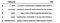Figure 1

Looks like long texts are there. We will see this in later sections. Here, the problem is: if a ‘text’ is given, we have to predict its ‘category’. Definitely, it is a multi-class text classification problem.

# Data Exploration & Visualisation

First, we will see how many categories are there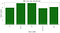Figure 2

So, there are 5 different categories. We can call these as ‘classes’. From the plot, it is clear that there is not that much skewness in the class distribution.

As a next step, we have to see what type of contents are there in the ‘text’ field of the dataset. For that, we have to clean the texts first.

A typical text cleaning process involves the following steps

1. Conversion to lowercase
2. Removal of punctuations
3. Removal of integers, numbers
4. Removal of extra spaces
5. Removal of tags (like <html>, <p> etc)
6. Removal of stop words (like ‘and’, ‘to’, ‘the’ etc)
7. Stemming (Conversion of words to root form)

We will use Python ‘gensim’ library for all text cleaning.

We can use this ‘clean_text’ function for doing the job.

Let’s print the first content of the text field of a record

`bbc_text_df.iloc[2,1]`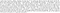Figure 3

After cleaning

`clean_text(bbc_text_df.iloc[2,1])`Figure 4

The text became little non-grammatical but it is required for understanding.

We will write a function for visualising ‘text’ contents as ‘Word Cloud’

We have to concatenate all texts and pass it to this function

`texts = ''for index, item in bbc_text_df.iterrows():    texts = texts + ' ' + clean_text(item['text'])    plot_word_cloud(texts)`

We will get now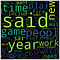Figure 5

Bigger words indicate ‘more frequent’. So, ‘year’, ‘time’, ‘peopl’ etc are the most frequent words.

Now, we will see more meaningful insights: ‘Word Cloud’ of ‘text’ for a particular ‘category’.

We will write a generic function for that

We will see the ‘Word Cloud’ for ‘category’ ‘tech’

`plot_word_cloud_for_category(bbc_text_df,'tech')`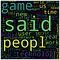Figure 6

So, for category ‘tech’ most frequent words are ‘peopl’, ‘techlog’ ‘game’ etc.

Similarly for ‘sport’

`plot_word_cloud_for_category(bbc_text_df,'sport')`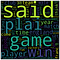Figure 7

Most frequent words are ‘plai’, ‘game’, ‘player’, ‘win’, ‘match’, ‘England’ etc

For the ‘politics’ category

`plot_word_cloud_for_category(bbc_text_df,'politics')`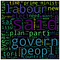Figure 8

‘govern’, ‘peopl’, ‘blair’, ‘countri’, ‘minist’ are the most frequent words.

Definitely, each category has some words which are distinguishing those from other categories. Or it may be like this : each ‘text’ is inferring some context in it which is determining its category

We need to do vector space analysis and use this in a model to get a confirmation of the above fact

# Vector Space Modelling & Building the Pipeline

Vector space modeling is essential for any NLP problem. We will try with the two most popular vector space models: ‘Doc2Vec’ & ‘Tf-Idf’. First, we will split the data into features and categories.

`df_x = bbc_text_df['text']df_y = bbc_text_df['category']`

## Doc2Vec

We will use Doc2Vec API of the ‘gensim’ library and write a generic ‘Doc2VecTransfoemer’

We will see how does the ‘Doc2Vec’ look like by applying this transformer

`doc2vec_trf = Doc2VecTransformer()doc2vec_features = doc2vec_trf.fit(df_x).transform(df_x)doc2vec_features`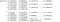Figure 9

So, it is a numerical representation of the text data. We can use these numerical features into any machine learning algorithm. We will try with LogisticRegression, RandomForest & XGBoost

For each of the cases we will do a 5-fold cross validation of the model with the dataset and test it. Accuracy score will be average of 5 folds.

Doc2Vec & LogisticRegression pipelineFigure 10

Accuracy came quite low !!

We will see other classifiers

Doc2Vec & RandomForest pipelineFigure 11

Not great again !!

Doc2Vec & XGBoost pipelineFigure 12

Not that much improvement.

‘Doc2Vec’ is not going good.

We will see ‘Tf-Idf’ vector space model

## Tf-Idf

We will write a similar transformer for ‘Tf-Idf’ also

We will see now, how does it transform the texts

`tfidf_transformer = Text2TfIdfTransformer()tfidf_vectors = tfidf_transformer.fit(df_x).transform(df_x)`

Printing its dimensions

`tfidf_vectors.shape`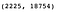Figure 13

There are total 18754 no of tokens

`print(tfidf_vectors)`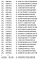Figure 14

Now, we will use this model in actual ML models

Tf-Idf & LogisticRegressionFigure 15

Tf-Idf is giving good accuracy !!

Tf-Idf & RandomForestFigure 16

Tf-Idf & XGBoostFigure 17

So, for the best comes at last !!

Off course, Tf-Idf & XGBoost combination will be our choice for solving this problem

# Explanation of the results

Though ‘Doc2Vec’ is a more advanced model in NLP rather than ‘Tf-Idf’, but still in our case, it is not giving proper results. We have tried with a linear, bagging & boosting based classifier respectively.

This reason can be explained. In our dataset, each ‘text’ field contains several words/tokens which are determining its category and the frequency of those is quite high. So making a context-sensitive model may over-complicate the situation or dilute this information. As the frequency of some tokens is high in some text categories, it is contributing large enough values in determining ‘Tf-Idf’. Also, ‘texts’ are domain-specific.

For example it is highly probable that ‘blair’ word will be present in ‘politics’ category rather than ‘sport’. So existence of this is contributing to ‘Tf-Idf’.

Furthermore, ‘Doc2Vec’ model is more suitable for very well written grammatically correct texts. In our case, texts are quite rough in nature.

One example of grammatically correct text could be ‘Wikipedia’ texts.

It is also proven in various examples and Data Scientist’s experiments that though ‘Tf-Idf’ model is inferior as compared to ‘Doc2Vec’, but still it gives better result while classifying very domain specific texts.

# Conclusion

This comes to end now. We tested with all combinations of classifiers and vector space models. Jupyter notebook for this can be found on GitHub.

Written by

## Towards AI

#### Towards AI, is the world’s fastest-growing AI community for learning, programming, building and implementing AI.

Welcome to a place where words matter. On Medium, smart voices and original ideas take center stage - with no ads in sight. Watch
Follow all the topics you care about, and we’ll deliver the best stories for you to your homepage and inbox. Explore
Get unlimited access to the best stories on Medium — and support writers while you’re at it. Just \$5/month. Upgrade